# 三种继承方式

### 2.继承和派生的目的

• 继承的目的：代码设计的重用
• 派生的目的：功能的扩展/更改

#### 3.继承的三种方式

• publicprivateprotected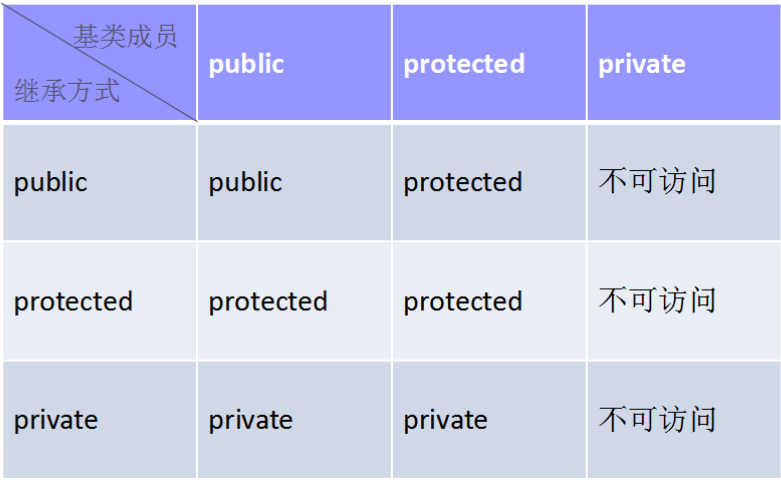class 派生名称：继承方式 基类1，继承方式 基类2，...{

}

• 一个实例：

	#include<iostream>
using namespace std;

//三种继承方式

class Base {//父类
private:
int b=1;
public:
void show() { cout << "Base's show" << endl; }
protected:
};

class Son1 :public Base {//子类1  public继承于Base
public:
//吸收基类成员的派生过程
//cout << "Base.b=" << b;  //虽然继承下了父类的private，但是不可以访问
};

class Son2 :private Base {//子类2    private继承于Base
public:
void show() {      //改造基类成员派生过程
Base::show();//访问父类public中的函数,因为和父类公有函数show同名，所以要通过作用域访问
//show();  //错误访问方式
cout << "Son2's show" << endl;
//cout << "Base.b=" << b;  //虽然继承下了父类的private，但是不可以访问
}
};

class Son3 :protected Base {//子类3    protected继承于Base
public:
void show3() {  //新添成员的派生过程
show();//也可以访问父类public中的函数
cout << "Son3's show" << endl;
//cout << "Base.b=" << b;  //虽然继承下了父类的private，但是不可以访问
}
};

int main() {
Son1 s1;
s1.show();//子类s1调用的是父类的show()
cout << "----------" << endl;
Son2 s2;
s2.show();//私有继承方式，所以不能调用父类的show(),  且是改造父类成员的派生过程，已经屏蔽了父类的show()
cout << "----------" << endl;
Son3 s3;
s3.show3();//保护继承方式，子类s3也不能调用到父类的show()

return 0;
}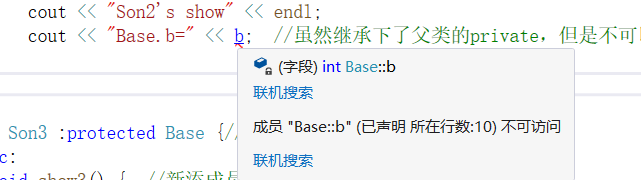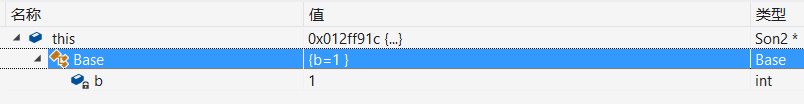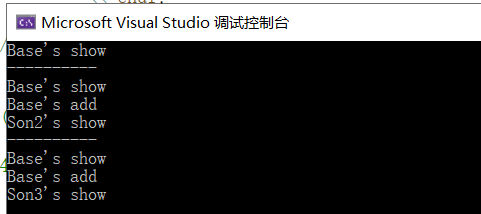• 也可以多继承，就上个例子来说，可以这样写:

  #include<iostream>
using namespace std;

class Base {//父类
private:
int b=1;
public:
void show() { cout << "Base's show" << endl; }
protected:
};
class Base1 { //父类
private:
public:
void pretend() { cout << "Base1's pretend" << endl; }
};

class Son4 :public Base, public Base1 {// 子类4   多继承
public:
void pretend4() { cout << "Son4's pretend" << endl; }
};
int main(){
Son4 s4;
s4.pretend();

s4.show();
return 0;
}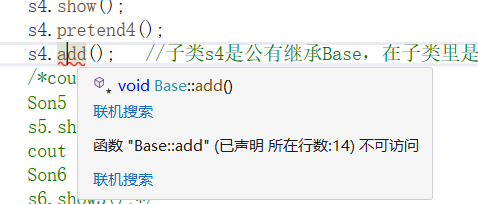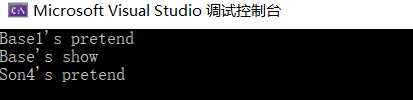• 多重继承

  #include<iostream>
using namespace std;

class Base {//父类
private:
int b=1;
public:
void show() { cout << "Base's show" << endl; }
protected:
};

class Son2 :private Base {//子类2    private继承于Base
public:

void pretend2() { cout << "Son2's pretend" << endl; }
};

class Son3 :protected Base {//子类3    protected继承于Base
public:
void show3() {  //新添成员的派生过程
show();//也可以访问父类public中的函数
cout << "Son3's show" << endl;
}
};

class Son5:public Son2{
public:
void show5() {
//show();   //因为直接父类是私有继承，后代即便是公有继承也无法访问"祖父"的成员函数
pretend2(); //调用直接父类的公有函数
}
};

class Son6 :public Son3 {
public:
void show6() {
show();//调用间接父类Base的公有函数

show3();//调用直接父类Son3的公有函数
}
};
int main() {
Son5 s5;
s5.show5();
cout << "----------" << endl;
Son6 s6;
s6.show6();
//s6.show(); //因为直接父类是保护继承，所以无法访问间接父类的公有函数
return 0;
}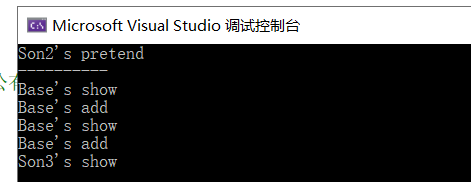• 继承中的构造函数和析构函数

  #include<iostream>
using namespace std;

class Base2 {
public:
Base2() { cout << "Base2 start" << endl; }//构造函数
~Base2() { cout << "Bsae2 end" << endl; }//析构函数
};

class Son7:public Base2 {
public:
Son7(){ cout << "Son7 start" << endl; }
~Son7() { cout << "Son7 end" << endl; }
};

int main() {
Son7 s7;
return 0;
}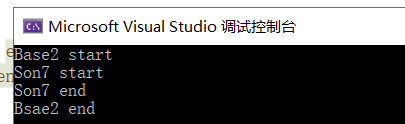## 总结：

• 子类拥有父类的所有成员变量和成员函数；（继承）
• 子类可以拥有父类没有的方法和属性；（派生）
• 子类对象可当作父类对象使用；（public继承方式）

posted on 2019-10-12 15:40  SailorMoon-z  阅读(196)  评论(0编辑  收藏  举报